# Multilevel HMM tutorial

Department of Methodology and Statistics, Utrecht University, Utrecht, the Netherlands

Abstract

With the package mHMMbayes you can fit multilevel hidden Markov models. The multilevel hidden Markov model (HMM) is a generalization of the well-known hidden Markov model, tailored to accdeommodate (intense) longitudinal data of multiple individuals simultaneously. Using a multilevel framework, we allow for heterogeneity in the model parameters (transition probability matrix and conditional distribution), while estimating one overall HMM. The model has a great potential of application in many fields, such as the social sciences and medicine. The model can be fitted on multivariate categorical or continous data, and include individual level covariates (allowing for e.g., group comparisons on model parameters). Parameters are estimated using Bayesian estimation utilizing the forward-backward recursion within a hybrid Metropolis within Gibbs sampler. The package also includes a function to simulate data and a function to obtain the most likely hidden state sequence for each individual using the Viterbi algorithm.

## Introduction

Hidden Markov models [HMMs; Rabiner (1989)] are a machine learning method that have been used in many different scientific fields to describe a sequence of observations for several decades. For example, translating a fragment of spoken words into text (i.e., speech recognition, see e.g. Rabiner 1989; Woodland and Povey 2002), or the identification of the regions of DNA that encode genes (i.e., gene tagging, see e.g., Krogh, Mian, and Haussler 1994; Henderson, Salzberg, and Fasman 1997; Burge and Karlin 1998). The development of this package is, however, motivated from the area of social sciences. Due to technological advancements, it becomes increasingly easy to collect long sequences of data on behavior. That is, we can monitor behavior as it unfolds in real time. An example here of is the interaction between a therapist and a patient, where different types of nonverbal communication are registered every second for a period of 15 minutes. When applying HMMs to such behavioral data, they can be used to extract latent behavioral states over time, and model the dynamics of behavior over time.
A quite recent development in HMMs is the extension to multilevel HMMs (see e.g., Altman 2007; Shirley et al. 2010; Rueda, Rueda, and Diaz-Uriarte 2013; Zhang and Berhane 2014; Haan-Rietdijk et al. 2017). Using the multilevel framework, we can model several sequences (e.g., sequences of different persons) simultaneously, while accommodating the heterogeneity between persons. As a result, we can quantify the amount of variation between persons in their dynamics of behavior, easily perform group comparisons on the model parameters, and investigate how model parameters change as a result of a covariate. For example, are the dynamics between a patient and a therapist different for patients with a good therapeutic outcome and patients with a less favorable therapeutic outcome?
With the package mHMMbayes, one can estimate these multilevel hidden Markov models. This tutorial starts out with a brief description of the HMM and the multilevel HMM. For a more elaborate and gentle introduction to HMMs, we refer to Zucchini, MacDonald, and Langrock (2016). Next, we show how to use the package mHMMbayes through an extensive example on categorical data, also touching on the issues of determining the number of hidden states and checking model convergence. Information on the used estimation methods and algorithms in the package is given in the vignette Estimation of the multilevel hidden Markov model.

## Hidden Markov models

Hidden Markov Models are used for data for which 1) we believe that the distribution generating the observation depends on the state of an underlying, hidden state, and 2) the hidden states follow a Markov process, i.e., the states over time are not independent of one another, but the current state depends on the previous state only (and not on earlier states) (see e.g., Rabiner 1989; Ephraim and Merhav 2002; Cappé 2005; Zucchini, MacDonald, and Langrock 2016). The HMM is a discrete time model: for each point in time $$t$$, we have one hidden state that generates one observed event for that time point $$t$$.
Hence, the probability of observing the current outcome $$O_t$$ is exclusively determined by the current latent state $$S_t$$:

$\begin{equation} Pr(O_{t} \mid \ O_{t-1}, O_{t-2}, \ldots, O_{1}, \ S_{t}, S_{t-1}, \ldots, S_{1}) = Pr(O_{t} \mid S_{t}). \end{equation}$

The probability of observing $$O_t$$ given $$S_t$$ can have any distribution, e.g., discrete or continuous. In the current version of the package mHMMbayes, only the categorical emission distribution is implemented.
The hidden states in the sequence take values from a countable finite set of states $$S_t = i, i \in \{1, 2, \ldots, m\}$$, where $$m$$ denotes the number of distinct states, that form the Markov chain, with the Markov property:

$\begin{equation} Pr(S_{t+1} \mid \ S_{t}, S_{t-1}, \ldots, S_{1}) = Pr(S_{t+1} \mid S_{t}). \end{equation}$

That is, the probability of switching to the next state $$S_{t+1}$$ depends only on the current state $$S_t$$. As the HMM is a discrete time model, the duration of a state is represented by the self-transition probabilities $$\gamma_{ii}$$, where the probability of a certain time t spent in state $$S$$ is given by the geometric distribution: $$\gamma_{ii}^{t-1}(1-\gamma_{ii})$$.
The HMM includes three sets of parameters: the initial probabilities of the states $$\pi_i$$, the matrix $$\mathbf{\Gamma}$$ including the transition probabilities $$\gamma_{ij}$$ between the states, and the state-dependent probability distribution of observing $$O_t$$ given $$S_t$$ with parameter set $$\boldsymbol{\theta}_i$$. The initial probabilities $$\pi_i$$ denote the probability that the first state in the hidden state sequence, $$S_1$$, is $$i$$:

$\begin{equation} \pi_i = Pr(S_1 = i) \quad \text{with} \sum_i \pi_i = 1. \end{equation}$

Often, the initial probabilities of the states $$\pi_i$$ are assumed to be the stationary distribution implied by the transition probability matrix $$\mathbf{\Gamma}$$, that is, the long term steady-state probabilities obtained by $$\lim_{T \rightarrow \infty} \mathbf{\Gamma}^T$$. The transition probability matrix $$\mathbf{\Gamma}$$ with transition probabilities $$\gamma_{ij}$$ denote the probability of switching from state $$i$$ at time $$t$$ to state $$j$$ at time $$t+1$$:

$\begin{equation} \gamma_{ij} = Pr(S_{t+1} = j \mid S_{t} = i) \quad \text{with} \sum_j \gamma_{ij} = 1. \end{equation}$

That is, the transition probabilities $$\gamma_{ij}$$ in the HMM represent the probability to switch between hidden states rather than between observed acts, as in the MC and CTMC model. The state-dependent probability distribution denotes the probability of observing $$O_t$$ given $$S_t$$ with parameter set $$\boldsymbol{\theta}_i$$. In case of the package, the state-dependent probability distribution is given by the categorical distribution, and the parameter set $$\boldsymbol{\theta}_i$$ is the set of state-dependent probabilities of observing categorical outcomes. That is,

$\begin{equation} Pr(O_t = o \mid S_t = i) \sim \text{Cat} (\boldsymbol{\theta}_i), \end{equation}$

for the observed outcomes $$o = 1, 2, \ldots, q$$ and where $$\boldsymbol{\theta}_i = (\theta_{i1}, \theta_{i2}, \ldots, \theta_{iq})$$ is a vector of probabilities for each state $$S = i, \ldots, m$$ with $$\sum \theta_i = 1$$, i.e., within each state, the probabilities of all possible outcomes sum up to 1.
We assume that all parameters in the HMM are independent of $$t$$, i.e., we assume a time-homogeneous model. In the vignette Estimation of the multilevel hidden Markov model we discuss three methods (i.e., Maximum likelihood, Expectation Maximization or Baum-Welch algorithm, and Bayesian estimation) to estimate the parameters of an HMM. In the package mHMMbayes, we chose to use Bayesian estimation because of its flexibility, which we will require in the multilevel framework of the model.

## Multilevel hidden Markov models

Given data of multiple subjects, one may fit the HMM to the data of each subject separately, or fit one and the same HMM model to the data of all subject, under the strong (generally untenable) assumption that the subjects do not differ with respect to the parameters of the HMM. Fitting a different model to each behavioral sequence is not parsimonious, computationally intensive, and results in a large number of parameters estimates. Neither approach lends itself well for a formal comparison (e.g., comparing the parameters over experimental conditions). To facilitate the analysis of multiple subjects, the HMM is extended by putting it in a multilevel framework.
In multilevel models, model parameters are specified that pertain to different levels in the data. For example, subject-specific model parameters describe the data collected within each subject, and group level parameters describe what is typically observed within the group of subjects, and the variation observed between subjects. In the implemented multilevel HMM, we allow each subject to have its own unique parameter values within the same HMM model (i.e., identical number and similar composition of the hidden states). Rather than estimating these subject-specific parameters individually, we assume that the parameters of the HMM are random, i.e., follow a given group level distribution. Within this multilevel structure, the mean and the variance of the group level distribution of a given parameter thus expresses the overall mean parameter value in a group of subjects and the parameter variability between the subjects in the group.
Multilevel HMMs have received some attention in the literature. In a frequentist context, Altman (2007) presented a general framework for HMMs for multiple processes by defining a class of Mixed Hidden Markov Models (MHMMs). These models are however, computationally intensive and due to slow convergence only suited for modeling a limited number of random effects. The approach of Altman has been translated to the Bayesian framework, which proved much faster as the time to reach convergence is decreased Zhang and Berhane (2014). In addition, the HMM in a Bayesian context is easier to adapt to a multilevel model, as the need for numerical integration is eliminated. Examples of the application of the multilevel HMM (within a Bayesian framework) are: Rueda, Rueda, and Diaz-Uriarte (2013) applied the model to the analysis of DNA copy number data, Zhang and Berhane (2014) to identify risk factors for asthma, Shirley et al. (2010) to clinical trial data of a treatment for alcoholism and Haan-Rietdijk et al. (2017) to longitudinal data sets in psychology.
In the tutorial, we use the following notation for the parameters in the multilevel HMM. The subject specific parameters are supplemented with the prefix $$k$$, denoting subject $$k \in \{1,2,\ldots,K\}$$. Hence, in the multilevel (Bayesian) HMM, the subject specific parameters are: the subject-specific transition probability matrix $$\boldsymbol{\Gamma}_k$$ with transition probabilities $$\gamma_{k,ij}$$, and the subject-specific emission distributions denoting subject-specific probabilities $$\boldsymbol{\theta}_{k,i}$$ of categorical outcomes within hidden state $$i$$. The initial probabilities of the states $$\pi_{k,j}$$ are not estimated as $$\pi_{k}$$ is assumed to be the stationary distribution of $$\boldsymbol{\Gamma}_k$$. The group level parameters are: the group level state transition probability matrix $$\boldsymbol{\Gamma}$$ with transition probabilities $$\gamma_{ij}$$, and the group level state-dependent probabilities $$\boldsymbol{\theta}_{i}$$. We fit the model using Bayesian estimation (i.e., a hybrid Metropolis Gibbs sampler that utilizes the forward-backward recursion to sample the hidden state sequence of each subject, see the vignette Estimation of the multilevel hidden Markov model).

## Using the package mHMMbayes

We illustrate using the package using the embedded categorical example data nonverbal. The data contains the nonverbal communication of 10 patient-therapist couples, recorded for 15 minutes at a frequency of 1 observation per second (= 900 observations per couple). The following variables are contained in the dataset:

• id: id variable of patient - therapist couple to distinguish which observation belongs to which couple.
• p_verbalizing: verbalizing behavior of the patient, consisting of 1 = not verbalizing, 2 = verbalizing, 3 = back channeling.
• p_looking: looking behavior of the patient, consisting of 1 = not looking at therapist, 2 = looking at therapist.
• t_verbalizing: verbalizing behavior of the therapist, consisting of 1 = not verbalizing, 2 = verbalizing, 3 = back channeling.
• t_looking: looking behavior of the therapist, consisting of 1 = not looking at patient, 2 = looking at patient. The top 6 rows of the dataset are provided below.

When we plot the data of the first 5 minutes (= the first 300 observations) of the first couple, we get the following: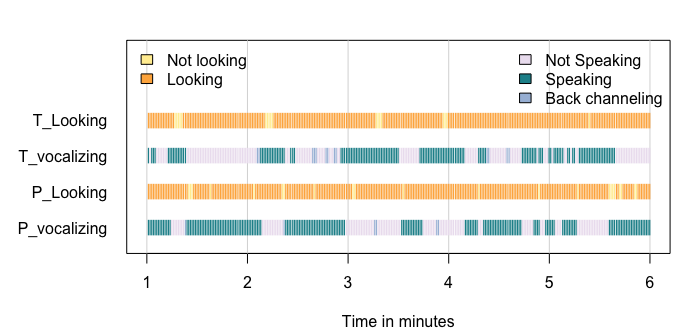We can, for example, observe that both the patient and the therapist are mainly looking at each other during the observed 5 minutes. During the first minute, the patient is primarily speaking. During the second minute, the therapists starts, after which the patient takes over while the therapist is back channeling.

### A simple model

To fit a simple 2 state multilevel model with the function mHMM, one first has to specify some general model properties and starting values:

library(mHMMbayes)
# specifying general model properties:
m <- 2
n_dep <- 4
q_emiss <- c(3, 2, 3, 2)

# specifying starting values
start_TM <- diag(.8, m)
start_TM[lower.tri(start_TM) | upper.tri(start_TM)] <- .2
start_EM <- list(matrix(c(0.05, 0.90, 0.05,
0.90, 0.05, 0.05), byrow = TRUE,
nrow = m, ncol = q_emiss), # vocalizing patient
matrix(c(0.1, 0.9,
0.1, 0.9), byrow = TRUE, nrow = m,
ncol = q_emiss), # looking patient
matrix(c(0.90, 0.05, 0.05,
0.05, 0.90, 0.05), byrow = TRUE,
nrow = m, ncol = q_emiss), # vocalizing therapist
matrix(c(0.1, 0.9,
0.1, 0.9), byrow = TRUE, nrow = m,
ncol = q_emiss)) # looking therapist

The first line of code loads the mHMMbayes package and the nonverbal data. Next we specify the general model properties: the number of states used is set by m <- 2, the number of dependent variables in the dataset used to infer the hidden states is specified by n_dep <- 4, and the number of categorical outcomes for each of the dependent variables is specified by q_emiss <- c(3, 2, 3, 2).

#### Starting values

The subsequent lines of code specify starting values for both the transition probability matrix and the emission distribution(s), which are given to the model in the argument start_val (see next piece of code). These starting values are used for the first run of the forward backward algorithm. Although the hidden states cannot be observed, one often has an idea for probable compositions of the states. In the example, we expect that there is a state in which the patient mostly speaks, and the therapist is silent, and a state during which the patient is silent and the therapists speaks. In addition, we expect that during both states, the therapist and the patient will be mainly looking at each other instead of looking away. One usually also has some (vague) idea on likely and unlikely switches between states, and the size of self-transition probabilities. In the example, we think a state will usually last quite some seconds, and thus expect a rather high self-transition probability. All these ideas can be used to construct a set of sensible starting values. Using sensible starting values increases convergence speed, and often prevents a problem called ‘label switching’. Hence, using random or uniform starting values is not recommended, and a default option to do so is not included in the package. Note that it is strongly advised to check model convergence and label switching. That is, one should check if the algorithm reaches the same solution when a set of different (but often conceptually similar) starting values are used, and if label switching is not a problem. See the section Checking model convergence and label switching for an example. See the vignette Estimation of the multilevel hidden Markov model) for more information on the forward backward algorithm and on the problem of label switching.

#### Prior distributions

As the estimation proceeds within a Bayesian context, a (hyper-)prior distribution has to be defined for the group level parameters, i.e., the group level emission and transition probabilities. Default, non-informative priors are used unless specified otherwise by the user. Below, we present some information on this. For a more elaborate explanation on the used (hyper-)prior distributions and their parameters, see the vignette Estimation of the multilevel hidden Markov model.
First of all, note that the prior distributions on the emission distribution and transition probabilities are not on the probabilities directly, but on the intercepts (and regression coefficients given that covariates are used) of the Multinomial regression model used to accommodate the multilevel framework of the data. Second, parameters do not each have their own independent prior distribution. As each row of the emission distribution matrix and transition probability matrix sum to one, the individual parameters of these rows are connected. Hence, each row is seen as a set of parameters which are estimated jointly, and each set of parameters has a multivariate prior distribution.
The sets of intercepts of the Multinomial regression model have a multivariate normal distribution. The (hyper-) prior for these intercepts thus consists of a prior distribution on the vector of means, and a prior distribution on the covariance matrix. The hyper-prior for the mean intercepts is a multivariate Normal distribution, with, as default, a vector of means equal to 0, and a parameter $$K_0$$ with which the covariance matrix is multiplied. Here, $$K_0$$ denotes the number of observations (i.e., the number of hypothetical prior subjects) on which the prior mean vector of zero’s is based. By default, $$K_0$$ is set to 1. The hyper-prior for the covariance matrix between the set of (state specific) intercepts has an Inverse Wishart distribution, for which the variance in the default setting equals 3 + $$m$$ - 1 for the transition probabilities and 3 + $$q$$ - 1 for the emission probabilities, and the covariance equals 0. The degrees of freedom of the Inverse Wishart distribution is set to 3 + $$m$$ - 1 for the transition probabilities and 3 + $$q$$ - 1 for the emission probabilities.
To specify user specific prior distributions, one uses the input option emiss_hyp_prior for the emission distribution and gamma_hyp_prior for the transition probabilities in the function mHMM. These input arguments take an object from the class mHMM_prior_emiss and mHMM_prior_gamma created by the functions prior_emiss_cat and prior_gamma, respectively. Both objects are a list, containing the following key elements:

• mu0, a lists containing the hypothesized hyper-prior mean values of the intercepts of the Multinomial logit model.
• K0, the number of hypothetical prior subjects on which the set of hyper-prior mean intercepts specified in mu0 are based.
• nu, degrees of freedom of the hyper-prior Inverse Wishart distribution on the covariance of the Multinomial logit intercepts.
• V, the variance-covariance of the hyper-prior Inverse Wishart distribution on the covariance of the Multinomial logit intercepts.

Note that K0, nu and V are assumed equal over the states. The mean values of the intercepts (and regression coefficients of the covariates) denoted by mu0 are allowed to vary over the states. All elements in the list either have the prefix gamma_ or emiss_, depending on which list they belong to. When specifying prior distributions, note that the first element of each row in the probability domain does not have an intercept, as it serves as baseline category in the Multinomial logit regression model. This means, for example, that if we would specify a model with 3 states, mu0 is a vector with 2 elements, K0 and nu contain 1 element and V is a 2 by 2 matrix.

#### Fitting the model

The multilevel HMM is fitted using the function mHMM:

# Run a model without covariate(s) and default priors:
set.seed(14532)
out_2st <- mHMM(s_data = nonverbal,
gen = list(m = m, n_dep = n_dep, q_emiss = q_emiss),
start_val = c(list(start_TM), start_EM),
mcmc = list(J = 1000, burn_in = 200))

The call to mHMM specifies the model with several arguments. The s_data argument specifies the input data used to infer the hidden states over time. The gen and start_val argument specify the general model properties and the starting values, as discussed above. The arguments needed for the MCMC algorithm are given in mcmc: J specifies the number of iterations used by the hybrid metropolis within Gibbs algorithm and burn_in specifies the number of iterations to discard when obtaining the model parameter summary statistics. The function mHMM returns an object of class mHMM, which has print and summary methods to see the results. The print method provides basic information on the model fitted. That is, the number of subjects in the dataset analyzed, the number of iterations and burn-in period, the average log likelihood over all subjects and model fit indices AIC, the number of states specified, and the number of dependent variables the states are based on:

out_2st
#> Number of subjects: 10
#>
#> 1000 iterations used in the MCMC algorithm with a burn in of 200
#> Average Log likelihood over all subjects: -1622.789
#> Average AIC over all subjects: 3273.579
#>
#> Number of states used: 2
#>
#> Number of dependent variables used: 4
#>
#> Type of dependent variable(s): categorical

The summary method provides information on the estimated parameters. That is, the point estimates of the posterior distribution for the transition probability matrix and the emission distribution of each of the dependent variables at the group level:

summary(out_2st)
#> State transition probability matrix
#>  (at the group level):
#>
#>              To state 1 To state 2
#> From state 1      0.930      0.070
#> From state 2      0.073      0.927
#>
#>
#> Emission distribution ( categorical ) for each of the dependent variables
#>  (at the group level):
#>
#> $p_vocalizing #> Category 1 Category 2 Category 3 #> State 1 0.010 0.972 0.018 #> State 2 0.812 0.033 0.155 #> #>$p_looking
#>         Category 1 Category 2
#> State 1      0.246      0.754
#> State 2      0.087      0.913
#>
#> $t_vocalizing #> Category 1 Category 2 Category 3 #> State 1 0.822 0.058 0.120 #> State 2 0.030 0.956 0.015 #> #>$t_looking
#>         Category 1 Category 2
#> State 1      0.045      0.955
#> State 2      0.272      0.729

The resulting model indicates 2 well separated states: one in which the patient is speaking and one in which the therapist is speaking. Looking behavior is quite similar for both the patient and the therapist in the 2 states. Information on the estimated parameters can also be obtained using the function obtain_gamma and obtain_emiss. These functions allow the user not only to inspect the estimated parameters at the group level, but for each subject individually as well, by specifying the input variable level = "subject":

# When not specified, level defaults to "group"
gamma_pop <- obtain_gamma(out_2st)
gamma_pop
#>              To state 1 To state 2
#> From state 1      0.930      0.070
#> From state 2      0.073      0.927

# To obtain the subject specific parameter estimates:
gamma_subj <- obtain_gamma(out_2st, level = "subject")
gamma_subj
#> $Subject 1 #> To state 1 To state 2 #> From state 1 0.945 0.055 #> From state 2 0.047 0.953 #> #>$Subject 2
#>              To state 1 To state 2
#> From state 1      0.937      0.063
#> From state 2      0.061      0.939
#>
#> $Subject 3 #> To state 1 To state 2 #> From state 1 0.968 0.032 #> From state 2 0.050 0.950 #> #>$Subject 4
#>              To state 1 To state 2
#> From state 1      0.940      0.060
#> From state 2      0.047      0.953
#>
#> $Subject 5 #> To state 1 To state 2 #> From state 1 0.942 0.058 #> From state 2 0.056 0.944 #> #>$Subject 6
#>              To state 1 To state 2
#> From state 1      0.939      0.061
#> From state 2      0.084      0.916
#>
#> $Subject 7 #> To state 1 To state 2 #> From state 1 0.930 0.070 #> From state 2 0.043 0.957 #> #>$Subject 8
#>              To state 1 To state 2
#> From state 1      0.933      0.067
#> From state 2      0.074      0.926
#>
#> $Subject 9 #> To state 1 To state 2 #> From state 1 0.948 0.052 #> From state 2 0.057 0.943 #> #>$Subject 10
#>              To state 1 To state 2
#> From state 1      0.960      0.040
#> From state 2      0.065      0.935

An additional option that the functions obtain_gamma and obtain_emiss offer is changing the burn-in period used for obtaining the summary statistics, using the input variable burn_in.

### Graphically displaying outcomes

The package includes several plot functions to display the fitted model and its parameters. First, one can plot the posterior densities of a fitted model, for both the transition probability matrix gamma and for the emission distribution probabilities. The posterior densities are plotted for the group level and the subject level simultaneously. For example, for the emission distribution for the variable p_vocalizing:

library(RColorBrewer)
Voc_col <- c(brewer.pal(3,"PuBuGn")[c(1,3,2)])
Voc_lab <- c("Not Speaking", "Speaking", "Back channeling")

plot(out_2st, component = "emiss", dep = 1, col = Voc_col,
dep_lab = c("Patient vocalizing"), cat_lab = Voc_lab)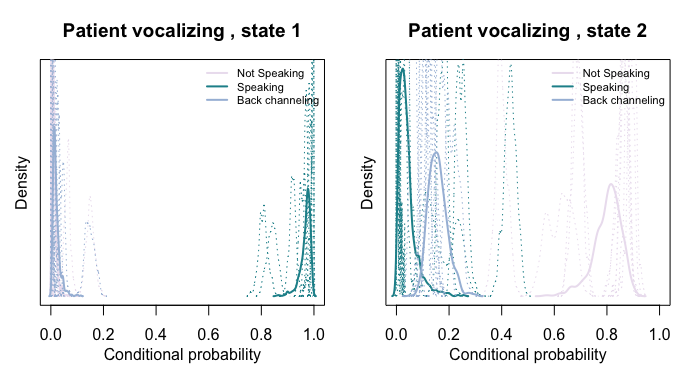Here, component specifies whether we want to visualize the posterior densities for the transition probability matrix gamma (component = "gamma") or for the emission distribution probabilities (component = "emiss"), when using component = "emiss" the input variable dep specifies which dependent variable we want to inspect (as the variable p_vocolizing is the first variable in the set, we set dep = 1), col specifies the colors to be used when plotting the lines, dep_lab denotes the label of the dependent variable we are plotting, and cat_lab denotes the labels of the categorical outcomes in the dependent variable. In the plot, the solid line visualizes the posterior density at the group level, while each of the dotted lines visualizes the posterior density of one subject.
Second, one can plot the transition probabilities obtained with the function obtain_gamma with a riverplot:

# Transition probabilities at the group level and for subject number 1, respectively:
plot(gamma_pop, col = rep(rev(brewer.pal(3,"PiYG"))[-2], each = m))
plot(gamma_subj, subj_nr = 1, col = rep(rev(brewer.pal(3,"PiYG"))[-2], each = m))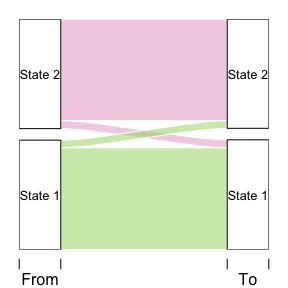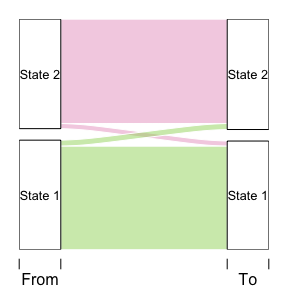Note that graphically displaying the transition probabilities becomes more informative as the number of states increase.

### Determining the number of hidden states

The first step in developing a HMM is to determine the number of states $$m$$ that best describes the observed data, and is a model selection problem. When modelling, for example, behavior, the task is to define the states by clusters of observed behavioral outcomes that provide a reasonable, theoretically interpretable, description of the data. We suggest using a combination of the Akaike Information Criterion (AIC) and the theoretical interpretability of the estimated states to choose between models1. In the example dataset, the 2, 3 and 4 state model result in an AIC of 3279, 3087, and 2959, respectively. According to model fit indices, the 4 state model is clearly the best model2. Let’s inspect the composition of the states for the 4 state model, and the transition probabilities:

summary(out_4st)
#> State transition probability matrix
#>  (at the group level):
#>
#>              To state 1 To state 2
#> From state 1      0.932      0.068
#> From state 2      0.073      0.927
#>
#>
#> Emission distribution ( categorical ) for each of the dependent variables
#>  (at the group level):
#>
#> $p_vocalizing #> Category 1 Category 2 Category 3 #> State 1 0.010 0.974 0.016 #> State 2 0.812 0.029 0.158 #> #>$p_looking
#>         Category 1 Category 2
#> State 1      0.243      0.757
#> State 2      0.087      0.913
#>
#> $t_vocalizing #> Category 1 Category 2 Category 3 #> State 1 0.822 0.058 0.120 #> State 2 0.029 0.956 0.015 #> #>$t_looking
#>         Category 1 Category 2
#> State 1      0.044      0.957
#> State 2      0.271      0.729

m <- 4
plot(obtain_gamma(out_4st), cex = .5, col = rep(rev(brewer.pal(5,"PiYG"))[-3], each = m))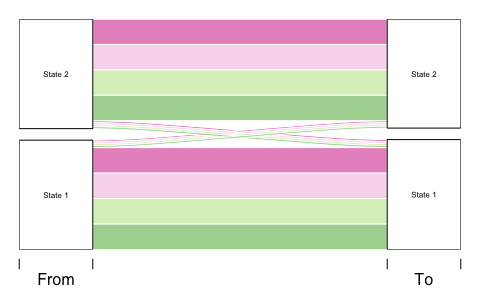We can see that we have a state in which the patient speaks and the therapist is silent (state 1), a state in which the patient is silent and the therapist speaks (state 2), a state in which both the patient and therapist speak (state 3) and a state in which the therapist speaks but does not look at the patient (in contrast to the looking behavior in all other states), and the patient is silent. In addition, all states are quite stable as the probability of remaining in the same state is above .6 for all states.

### Determining the most likely state sequence

Given a well-fitting HMM, it may be of interest to determine the actual sequence, or order of succession, of hidden states that has most likely given rise to the sequence of outcomes as observed in a subject. One can either use local decoding, in which the probabilities of the hidden state sequence are obtained simultaneously with the model parameters estimates, or the well-known Viterbi algorithm (Viterbi 1967; Forney Jr 1973). In local decoding, the most likely state is determined separately at each time point $$t$$, in contrast to the Viterbi algorithm in which one determines the joint probability of the complete sequence of observations $$O_{1:T}$$ and the complete sequence of hidden states $$S_{1:T}$$.
In the package, local decoding can be achieved by saving the sampled hidden state sequence at each iteration of the Gibbs sampler, by setting the input variable return_path = TRUE for the function mHMM. This will result in very large output files, however. Global decoding can be performed by using the function vit_mHMM:

state_seq <- vit_mHMM(out_2st, s_data = nonverbal)
#> Please note that the output format is changed from wide to long format to facilitate aditionally returning the state probabilities, see the section 'Value' in the help file for more information.
#>   subj state
#> 1    1     1
#> 2    1     1
#> 3    1     1
#> 4    1     1
#> 5    1     1
#> 6    1     1

The function returns the hidden state sequence for each subject in a matrix, where each row represents a point in time and each column represents a subject. We can inspect the obtained hidden state sequence by for example plotting it together with the observed data. Below, the first 5 minutes of the first couple is plotted again, with the addition of the estimated state sequence: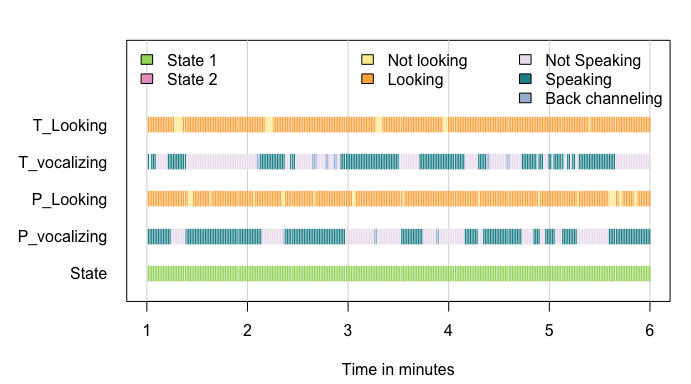### Checking model convergence and label switching

When using Bayesian estimation procedures, it is strongly advised to check model convergence and label switching. That is, one should check if the algorithm reaches the same solution when a set of different (but often conceptually similar) starting values are used, and if label switching is not a problem. With label switching, the label (i.e., which state represents what) ordering of the states switches over the iterations of the estimation algorithm. For example, what started out as state 1, now becomes state 2. One can check model convergence and label switching visually by inspecting the trace plots of parameters of a set of identical models that used varying starting values. Trace plots are plots of the sampled parameter values over the iterations. First, we fit the model with 2 states again, but with different starting values:

# specifying general model properties
m <-2
n_dep <- 4
q_emiss <- c(3, 2, 3, 2)

# specifying different starting values
start_TM <- diag(.8, m)
start_TM[lower.tri(start_TM) | upper.tri(start_TM)] <- .2
start_EM_b <- list(matrix(c(0.2, 0.6, 0.2,
0.6, 0.2, 0.2), byrow = TRUE,
nrow = m, ncol = q_emiss), # vocalizing patient
matrix(c(0.4, 0.6,
0.4, 0.6), byrow = TRUE, nrow = m,
ncol = q_emiss), # looking patient
matrix(c(0.6, 0.2, 0.2,
0.2, 0.6, 0.2), byrow = TRUE,
nrow = m, ncol = q_emiss), # vocalizing therapist
matrix(c(0.4, 0.6,
0.4, 0.6), byrow = TRUE, nrow = m,
ncol = q_emiss)) # looking therapist

# Run a model identical to out_2st, but with different starting values:
set.seed(9843)
out_2st_b <- mHMM(s_data = nonverbal,
gen = list(m = m, n_dep = n_dep, q_emiss = q_emiss),
start_val = c(list(start_TM), start_EM),
mcmc = list(J = 1000, burn_in = 200))

The group level parameter estimates of the emission probabilities and the transition probability matrix at each iteration of the estimation algorithm are stored in the objects emiss_prob_bar and gamma_prob_bar, respectively. The subject level parameter estimates are stored in the object PD_subj, where PD is an abbreviation for posterior density. If we, for example, want to inspect the trace plots for the emission probabilities for looking behavior of the patient at the group level, we use the following code:

par(mfrow = c(m,q_emiss))
for(i in 1:m){
for(q in 1:q_emiss){
plot(x = 1:1000, y = out_2st$emiss_prob_bar[][,(i-1) * q_emiss + q], ylim = c(0,1.4), yaxt = 'n', type = "l", ylab = "Transition probability", xlab = "Iteration", main = paste("Patient", Look_lab[q], "in state", i), col = "#8da0cb") axis(2, at = seq(0,1, .2), las = 2) lines(x = 1:1000, y = out_2st_b$emiss_prob_bar[][,(i-1) * q_emiss + q], col = "#e78ac3")
legend("topright", col = c("#8da0cb", "#e78ac3"), lwd = 2,
legend = c("Starting value set 1", "Starting value set 2"), bty = "n")
}
}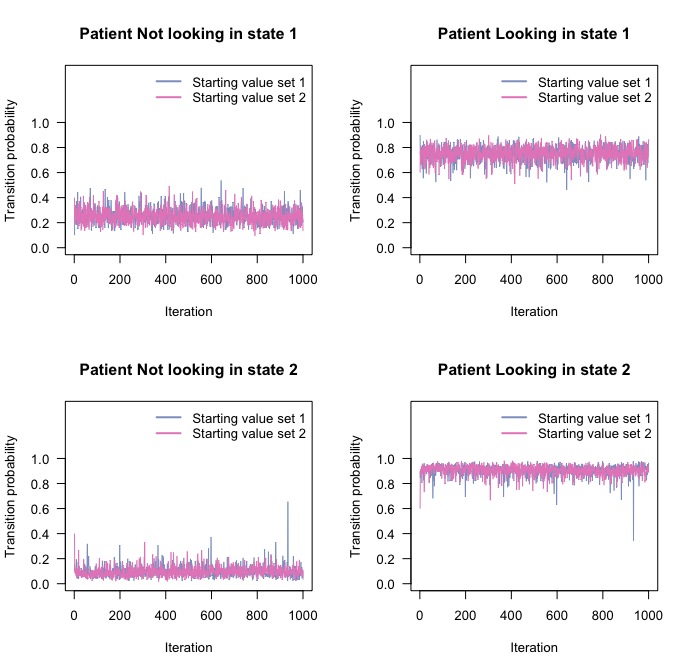It can be observed that the parameter estimates converge to the same parameter space, and that the chains mix well. Also, there is no evidence of label switching.

1. Note that the likelihood ratio test, commonly used to compare nested models, cannot be used in case of the HMM (i.e., the difference in the log-likelihoods between models is not $$\chi^2$$ distributed Rydén (2008)).↩︎

2. We note, however, that the AIC approximates the posterior distribution of the parameters by a Gaussian distribution, which might not be appropriate for models including parameters on the boundary of the parameter space (e.g., close to 0 or 1 in case of probability estimates), or for small data sets, as exemplified by Scott (2002). Model selection is therefore not a straightforward procedure in the context of HMM, and the choices remain subjective.↩︎